Published: 07 October 2016

# The approach to building the algorithm for controlling rotor motion in a hybrid mechatronic bearing

Roman Polyakov1
Maxim Bondarenko2
Denis Shutin3
Leonid Savin4
1, 2, 3, 4Orel State University, Orel, Russia
Corresponding Author:
Roman Polyakov
Views 29

#### Abstract

The paper describes the approach to building the algorithm for controlling the rotor motion in a hybrid mechatronic bearing. Such bearings include a rolling bearing, a gas foil bearing, an electromagnetic and a piezo actuator. Fuzzy logic techniques are used in the proposed algorithm. Its main aim is to minimize the deviation of the rotor position in the bearing from the equilibrium position. It results in reducing the vibrational activity of the rotor-bearing system and reducing the friction losses.

## 1. Introduction

Increasing power density of machinery causes tightening the requirements to operability and reliability of bearings. Operation of rotating machinery is always accompanied with the appearance of unwanted vibrations of rotor caused by imbalance, lubricant pressure pulsation and other reasons. Such vibrations cause the negative effect on quality of the machinery operation. In some cases, they can be dangerous due to the increased load on bearings.

The quality of operation of rotating machinery can be improved by applying the special types of bearings, e.g. hybrid bearings that include a rolling bearing and a fluid-film bearing in one unit. The first studies on hybrid bearings appeared in 1960s-1970s [1, 2]. In the 1980s NASA (USA) launched several research programs on hybrid bearings for cryogenic turbo pumps [3, 4]. The progress in electronics and mechatronics at the beginning of the XXI century resulted in development of active hybrid bearings that provide better characteristics than traditional passive bearings. So, the present paper is devoted to the hybrid mechatronic bearing.

## 2. Design of the hybrid mechatronic bearing

The proposed design of the hybrid mechatronic bearing  is illustrated in the Fig. 1: the bearing consists of the housing 1, rolling bearing 2 mounted in the housing. The sleeve 3 and metal plates 4 are mounted in the rolling bearing 2. The plates provide centering of the shaft 5. The electromagnetic coils 6 are mounted around the inner diameter of the housing. Piezo actuators 7 mounted on the ring 8 are used for locking the inner ring of the rolling bearing during the main operation mode.

The device operates as follows: firstly, the load is transferred from the shaft 5 to the housing 1 through the metal plates 4 and rolling elements in the rolling bearing 2. As the rotation speed increases, extra voltage is applied to electromagnetic coils 6 and piezo actuators 7. The arising electromagnetic field turns the plates 4 from the surface of the shaft 5 which creates an air gap. Gas-dynamic force that arises in the gap centers the shaft and carries the external load. The inner ring of the rolling bearing 2 is locked with piezo actuators 7 so that the rolling bearing 2 stops revolving. When the rotation speed decreases the whole process is reversed. Herewith rotor movement stability is improved due to the increased damping properties on account of the metal plates influence.

Fig. 1Hybrid mechatronic bearing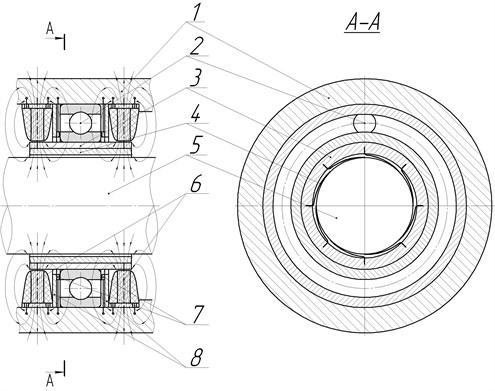## 3. Control system and algorithm

Development of intellectual control systems for rotating machinery is a labor-consuming process, especially in terms of combining the mathematics, algorithms, software and practical implementation of such systems. With that, the intellectual systems are able to make the rational control decisions to provide the machine operability in conditions of uncertainty [6-8].

The main advantages of applying active control systems to bearing of rotating machinery are as follows:

1) ability of adaptation of a rotor-bearing system to the changing operation conditions due to the rapid change of dynamic characteristics of a bearing (rigidity, damping);

2) ability of implementation of algorithms of rotor vibrations reduction using the built-in mechatronic system including sensors and actuators;

3) sensor system allows to track the parameters of a hybrid bearing in real-time mode, detect the malfunctions in the rotor-bearing system at the early stages and thus avoid emergency situations .

The rotor-bearing unit is a complicated object for control process due to the fact that many parameters vary during its operation, and many of them significantly influence on the control process. Thereby the special control methods are required to provide the stability of rotor at different operation modes. The artificial intelligence methods have significant advantages in such application. They allow to avoid using hard analytical relations in control laws and keep operability of the controlled system in complicated conditions.

Algorithmization of intellectual control in hybrid mechatronic bearings can be divided into two basic stages:

1) evaluation of the processes in the rotary system, including recognition of rotor’s oscillation trajectories as graphical objects and determining its significant features, primarily the amplitudes;

2) generation of control actions based on the obtained system parameters and implementation of the control strategy consisting in generation of the specific sets of control actions.

The algorithms of recognition of the rotor’s oscillations features should include the analysis of sets of rotor movements in the bearing that fully and uniquely characterize the processes in a rotor-bearing system. The result of such analysis is the data about the oscillations in the system, including its type and parameters, primarily amplitude. Analysis of variation of oscillation amplitude allow estimating the condition of the rotor-bearing system in general. This data is also a basis for making a decision about the required control signal.

Control algorithms in the considered hybrid mechatronic bearing are built in terms of overcoming the frequency zones that are most difficult from the standpoint of operability of bearings. The operability and reliability of a rolling bearing (RB) is limited at high frequencies. The operability and reliability of a gas foil bearing (GFB) is limited at low frequencies. Increasing the rotation frequency leads to redistribution of the load capacity between the RB and the GFB. However, the redistribution process is extended in time, and the load smoothly moves from the low-frequent RB to the high-frequent GFB, so for some period of time the components of a hybrid bearing operate in non-optimal mode. It leads to the premature wear. Moreover, in passive hybrid bearings at high rotation frequencies the load is not fully removed from the RB. It also leads to its accelerated wear. This problem is solved in a mechatronic hybrid bearing. In this bearing the distribution of load and rotation speed is actively controlled.

So, the basic operation of hybrid mechatronic bearing can be described with the variable $\chi$ with changing rate from 0 to 1. It shows the load/speed distribution between the RB and GFB. $\chi$ = 0 means that the all the load is acting on the low-frequent RB, and vice versa. Thus, the global control problem is to find the $\chi$ value that best suits the current mode of the rotor-bearing system operation. Let us describe the optimal algorithm of loads distribution and introduce the functional θ describing the optimality of operation of a hybrid bearing’s component under certain conditions. The value $\theta$ = 1 will mean that it is optimal to apply 100 % of the load to the considered component, and vice versa. Within the range from 0 to 1 the optimal load changes proportionally. The RB operates mostly efficiently and with minimal wear at low rotation speed, so at the low frequencies ${\theta }_{RB}$ = 1. When rotation speed increases, ${\theta }_{RB}$ decreases to zero. For the GFB the situation is reversed, they have minimal wear and best dynamic characteristics at high-frequencies. So, we get the graphical representation of the optimality functions as it is shown in Fig. 2.

Fig. 2Zones of optimal operation of the hybrid bearing components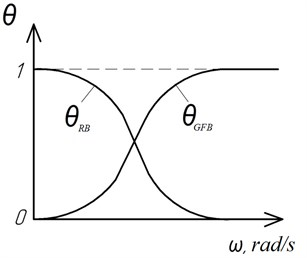The obtained relations ${\theta }_{RB}\left(\omega \right)$ and ${\theta }_{GFB}\left(\omega \right)$ have the form and the meaning similar to the membership functions being used in fuzzy logic. Also, fuzzy logic techniques can work well in complicated nonlinear system. So, let us apply the experience in usage of RB and GFB and create a fuzzy logic system for controlling the process of operation of rotor systems with hybrid mechatronic bearings.

Let us use the rotation frequency variable ${\omega }^{*}$ (“Rotation frequency”) as the fuzzy variable. It will be used as the basic variable for generating the control signal. Let us define a term set for ${\omega }^{*}$:

1
${\omega }^{\mathrm{*}}=\left\{\text{"}\text{Zero}\text{",}\text{}\text{"}\text{Low}\text{",}\text{}\text{"}\text{Medium}\text{",}\text{}\text{"}\text{High}\text{-}\text{Medium}\text{",}\text{}\text{"}\text{High}\text{"}\right\}.$

It is also necessary to take into consideration the load acting on the rotor-bearing system in order to provide the good quality of its operation on hybrid mechatronic bearings. The load factor can be formalized with the load coefficient $q$. Its value shows how high and dangerous the current load level in the system is. Q ratio is evaluated based on the real-time data obtained from the rotor-bearing system. Due to the fact that the hybrid mechatronic bearing consists of several parts $q$ coefficient should also be composite:

2
$q={q}_{RB}+{q}_{FFB}.$

The load acting on RB can be considered on the basis of the vibrational parameters of its operation because RB has low damping capacity, high rigidity and therefore it largely transfers the rotor vibrations to the housing. These parameters are usually measured with the sensors (accelerometers) mounted on the bearing housing. The load acting on GFB can be considered on the basis of the amplitude of rotor movements within the GFB lubricant layer in its gap.

For taking into consideration the load in the hybrid mechatronic bearing control system let us introduce one more fuzzy variable ${q}^{*}$ “Load” and define a term set for it:

3
${q}^{\mathrm{*}}=\left\{\text{"}\text{Minimal}\text{",}\text{"Valid}\text{",}\text{"High}\text{",}\text{"VeryHigh}\text{"}\right\}.$

The variable ${\chi }^{*}$ “Loads ratio” will be used as the output of the fuzzy controller. It shows the required ratio of the loads that should be perceived by the components of the hybrid mechatronic bearing (RB and GFB) at the current time. Physically this ratio is implemented in the bearing by the actuator that redistributes the load between the RB and the GFB. The term set for ${\chi }^{*}$ is as follows:

4
${\chi }^{\mathrm{*}}=\left\{\text{"}\text{OnlyLF}\text{(}\text{LF}\text{)",}\text{}\text{"MoreLF}\text{,}\text{lessHF}\text{(}\text{LF}\text{>}\text{HF}\text{)",}\right\$
$\text{"LF}\text{&}\text{HFthesame}\text{(}\text{LF}\text{=}\text{HF}\text{)",}\text{}\text{"}\text{LessLF}\text{,}\text{moreHF}\text{(}\text{LF}\text{<}\text{HF}\text{)",}\text{}\text{"}\text{OnlyHF}\text{(}\text{HF}\text{)"}}.$

The membership functions for fuzzy variables ${\omega }^{\mathrm{*}}$, ${q}^{\mathrm{*}}$ and ${\chi }^{\mathrm{*}}$ are shown in the Fig. 3. The upper limit of the range of the variables ${\omega }^{\mathrm{*}}$ (Fig. 3(a)) and ${q}^{\mathrm{*}}$ (Fig. 3(b)) are not specified because it depends on particular characteristics of the considered system. The membership functions for ${\chi }^{\mathrm{*}}$ (Fig. 3(c)) are limited from both sides.

Fig. 3Membership functions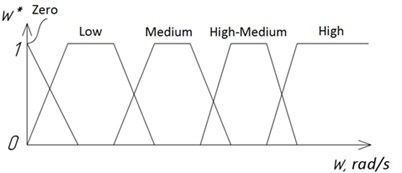a)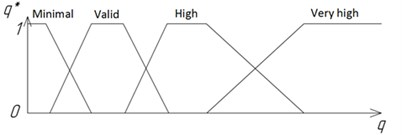b)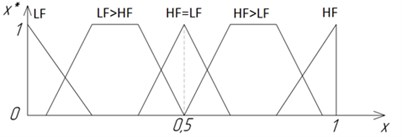c)

The fuzzy rules for determining the value of the fuzzy variable ${\chi }^{\mathrm{*}}$ are presented as a Table 1. After that the defuzzification process is used to find the value the parameter $\chi$. Finally, $\chi$ is used to evaluate the value of the control signal sent to the bearing’s actuator.

Table 1Fuzzy rules for determining χ*

 ${q}^{\mathrm{*}}$ “Minimal” “Valid” “High” “Very high” ${\omega }^{\mathrm{*}}$ “Zero” LF LF LF LF “Low” LF>HF LF>HF LF LF “Medium” HF=LF HF=LF HF=LF HF>LF “High-medium” HF HF>LF HF>LF HF>LF “High” HF HF HF HF

The rules consider that the high-frequency part of the hybrid mechatronic bearing (GFB) bears more load with increasing the rotation speed (parameter $\chi$ increases). When the load level is non-zero, but is moderate, the $\chi$ value increases mostly evenly. Under the minimal load the probability of mechanical contact of the rotor and the GFB housing is low. In this case the switching process from RB to GFB is sharper for providing higher wear resistance of the system. Under the high and very high load it is expedient to use mostly the low-frequency RB due to its high rigidity. It allows avoiding damaging the GFB.

## 4. Modeling results

The dynamic behavior of the rotor in the hybrid mechatronic bearing was studied using the mathematical model of a multi-mass rigid rotor . The RB was modeled using the Hertz contact theory, such modeling of a RB is considered, e.g., in . The GFB was modeled using the numerical solution of Navier-Stockes equations, the same approach is shown in . The rotor operation process has been modeled for active hybrid mechatronic bearing (Fig. 4(a)) and for passive hybrid bearing (Fig. 4(b)) with the same constructive and operational parameters. The result of modeling is shown as the rotor trajectories in the bearing.

Fig. 4Modeling of operation of the rotor-bearing system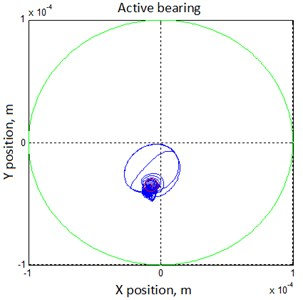a)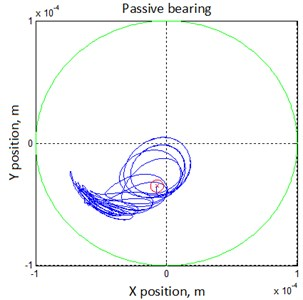b)

The Fig. 4 shows that using a hybrid mechatronic bearing allows reducing rotor oscillations by 25…40 %. Also, it allows in some cases eliminating the non-synchronous oscillations that cause big loads on the elements of the rotating machinery.

## 5. Conclusion

The article substantiates the facilities of active control of rotor motion to ensure the proper margin of vibration resistance. The main features of the developed algorithm are the heightened stability during operation at different rotation frequencies and the possibility of automatic adjustment of control parameters at different combinations of geometrical and working parameters of rolling bearing, sliding bearings and actuators.

Active control of the rotor position in the bearing allows setting its position arbitrarily. This effect can be used for the further optimization of operational parameters of a rotor-bearing system. So, some researches show that there is a minimum of friction in fluid-film and gas bearings at the rotor relative eccentricity about 0,7 . It is possible to use the control system of a hybrid mechatronic bearing for controlling the relative eccentricity of the rotor. Thus, mechatronic hybrid bearings are able not only to reduce vibrational activity of the rotor-bearing system, but also reduce the friction losses during its operation.

31 July 2016
Accepted
03 September 2016
Published
07 October 2016
SUBJECTS
Vibration generation and control
Keywords
hybrid bearing
rolling bearing
gas foil bearing
active control
mechatronic bearing
friction losses
energy efficiency
Acknowledgements

The research was implemented in the framework of the Russian Scientific Foundation Project No. 16-19-00186.